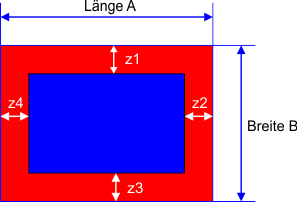### Planning Guide

Here you will find the calculation basis for the required heating mats

1. Measure the dimensions of the room ( A, B )2. Specify the border area ( z1, z2, z3, z4 )

• Recommendation: edge zone of 60 cm as storage space for furniture, as heating is not required under furniture
• Minimum distance to the wall is however 5 cm3. Calculate the area to be heated ( F )

• determine the new length and width
A1 = A - z2 - z4 und B1 = B - z1 - z3
• heatable surface: F = A1 x B14. Choose your heating mat corresponding to the heatable area F from our shop

Calculation example:

Room length = 6m

Room width = 4m

border zone of 0,6m each

Length A1 = 6m - 0,6m - 0,6m

Length B1 = 4m - 0,6m - 0,6m

Heatable area = 13,44m²